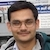# Relative velocity of trees when looking through the window of a moving trainBy

When looking through the window of a moving train, the nearby trees/objects appear to move in the opposite direction. The faraway trees appears to be stationary. This can be explained by the concept of relative velocity and perception of motion by the eye.

Let the train moves with a velocity $\vec{v}_A$ (relative to the ground). The velocity of the tree is $\vec{v}_B=\vec{0}$. The velocity of the tree relative to the train is $\vec{v}_{B/A}=\vec{v}_B-\vec{v}_A=-\vec{v}_A$. Thus, for a person sitting in the moving train, nearby trees appear to move in opposite direction.

The eye perceives velocity as the angular velocity of the line of sight (the line from eye to the object). The angular velocity of faraway objects is very small which makes faraway trees appear as stationary. It is similar to the illusion as if the moon is following us.

## Assertion Reasoning Problem From IIT JEE 2008

Problem:

Statement 1: For an observer looking out through the window of a fast moving train, the nearby objects appear to move in the opposite direction to the train, while the distant objects appear to be stationary.

Statement 2: If the observer and the object are moving at velocities $\vec{v}_1$ and $\vec{v}_2$ respectively with reference to a laboratory frame, the velocity of the object with respect to the observer is $\vec{v}_2-\vec{v}_1$.

1. Statement 1 is true, statement 2 is true; statement 2 is a correct explanation for statement 1.
2. Statement 1 is true, statement 2 is true; statement 2 is not a correct explanation for statement 1.
3. Statement 1 is true, statement 2 is false.
4. Statement 1 is false, statement 2 is true.
Solution:

Both, statement 1 and statement 2 are true but statement 2 is not a correct explanation of statement 1. The relative velocity of the nearby, as well as the far-off objects, is the same.The eye perceive the motion of an object through motion of its image on retina. It sees motion as angular velocity of the line of sight.

The explanation has to do with how human mind/eye perceive the motion. Take a simple situation of two point objects $\mathrm{O_1}$ and $\mathrm{O_2}$ (see figure). The images $\mathrm{I_1}$ and $\mathrm{I_2}$ formed on the retina of eye are point images. As the object moves, the image on the retina also moves. The distance moved by the image on the retina is perceived as motion/velocity. Since eye is a lens with distance of retina/screen w.r.t. lens almost fixed, the angular displacement of the image in a given time is perceived as velocity. For same relative velocity of objects $\mathrm{O_1}$ and $\mathrm{O_2}$, angular displacement of nearby object ($\theta_1$ in the figure) is more than the angular displacement of the far-off object ($\theta_2$). Hence nearby objects appear to move faster.